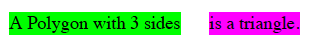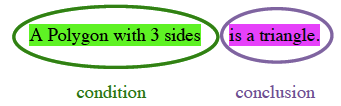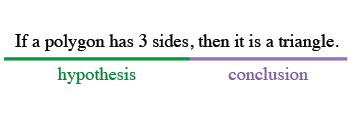Home > CCG > Chapter 3 > Lesson 3.1.3 > Problem3-33

3-33.

Rewrite the statements below into conditional (“If …, then …”) form. Homework Help ✎

Try separating each statement into hypothesis and conclusion.

Rewrite the sentence.1. Lines with the same slope are parallel.

If the lines have the same slope, then they are parallel.

2. A vertical line has undefined slope.

3. The lines with slopes $\frac { 2 } { 3 }$ and $-\frac{3}{2}$ are perpendicular.